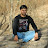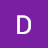water analogy for voltage current and resistanceMrNams Published on Nov 25, 2017
1597 views

Share, Support, Subscribe!!! Subscribe: https://goo.gl/mqbdai Facebook: https://www.facebook.com/MrNamsOfficial Twitter: https://twitter.com/MrNams Hello friends, Hope you all doing well! In this video, we shall understand voltage, current, register and Ohm’s Law through water-and-pipe analogy. Hash tags #voltage stabilizer #electric motor #electric current #voltage regulator #ohm's law #analogy #voltage current and resistance This video may help to understand 1) Components Semiconductor: FET / BJT, IC's, Digital, Analog, Interface, Mixed Signal, Glue Logic Searches Passive: Capacitors, Inductors, LED's, Oscillators, Resistors, Sensors, Transformers / Chokes Mechanical: Batteries, Connectors, Chassis, EMI Shielding, Fans, Fuses, Heat Sinks, IC Sockets, Motors, Nuts / Bolts, Switches, Relays, Wires / Cable 2) Terms AC – Alternating Current, Resistor, Capacitor, Inductor, Electrical & electronic symbols direct current (DC, also dc), Amperage – Amps/Amperes/Ampacity/Rated Amperage, AWG – American Wire Gauge, Cable, Cable Harness, CE – Conformite Europeene, Conductor, Connector, 3)Laws: Coulomb's law, DC circuit laws, Kirchhoff's laws (KVL / KCL), Ohm's law, Voltage divider rule, Ohm’s Law, Kirchhoff’s Laws, Thévenin’s Theorem, Norton’s Theorem, Thévenin and Norton Equivalence, Superposition Theorem, Reciprocity Theorem, Compensation Theorem, Millman’s Theorem, Joule’s Law, Maximum Power Transfer Theorem, Star-Delta Transformation, Delta-Star Transformation What is voltage: Voltage, also called electromotive force, is a quantitative expression of the potential difference in charge between two points in an electrical field. Voltage, electric potential difference, electric pressure or electric tension (formally denoted ?V or ?U, but more often simply as V or U, for instance in the context of Ohm's or Kirchhoff's circuit laws) is the difference in electric potential between two points per unit electric charge. Voltage is the pressure from an electrical circuit’s power source that pushes charged electrons (current) through a conducting loop, enabling them to do work such as illuminating a light. In brief, voltage = pressure, and it is measured in volts (V). The term recognizes Italian physicist Alessandro Volta (1745-1827), inventor of the voltaic pile—the forerunner of today’s household battery. In electricity’s early days, voltage was known as electromotive force (emf). This is why in equations such as Ohm’s Law, voltage is represented by the symbol E. What is Electric Current: Electric current is the rate of charge flow past a given point in an electric circuit, measured in Coulombs/second which is named Amperes. In most DC electric circuits, it can be assumed that the resistance to current flow is a constant so that the current in the circuit is related to voltage and resistance by Ohm's law. The standard abbreviations for the units are 1 A = 1C/s What is Resistance: Resistance is the opposition that a substance offers to the flow of electric current. It is represented by the uppercase letter R. The standard unit of resistance is the ohm, sometimes written out as a word, and sometimes symbolized by the uppercase Greek letter omega: Greek letter omega When an electric current of one ampere passes through a component across which a potential difference (voltage) of one volt exists, then the resistance of that component is one ohm. (For more discussion of the relationship among current, resistance and voltage, see Ohm's law.) What is Ohm's law: Ohm's law states that the current through a conductor between two points is directly proportional to the voltage across the two points. Introducing the constant of proportionality, the resistance, one arrives at the usual mathematical equation that describes this relationship I=V/R, where I is the current through the conductor in units of amperes, V is the voltage measured across the conductor in units of volts, and R is the resistance of the conductor in units of ohms. More specifically, Ohm's law states that the R in this relation is constant, independent of the current. THANKS FOR WATCHING. If you enjoyed video, please do like, share and comment. Please SUBSCRIBE to follow new Uploads https://goo.gl/mqbdai WARNING: Always be safe, everything you do is at YOUR OWN RISK! I shall not be liable for any injuries, loss or damages claimed to be caused by watching the contents either. #YouTubeRewind Rewind Rewind 2016 #Rewind YouTubeRewind 2018 Happy new year 2018
Comments ( 2)
•Sagar Velhal Published on Nov 28, 2017 very nice explanation 2
•Dhiraj Tikore Published on Nov 28, 2017 👍👍👍 1